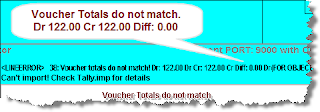## Tuesday, November 4, 2008

### Tally Error: Voucher Totals do not match

"Voucher Totals do not match. Dr 122.00 Cr 122.00 Diff 0.00"Surprised. Even though the Debit and Credit figures shown in the error are same, Tally Software still shows Dr and Cr mismatch.

Cause of the Problem:-
It is likely that the Excel sheet that you are using contains some FORMULA's. Though the display format (may) have been set to 2 decimals, MS-Excel internally does not round it off to 2 decimals until you use the =ROUND() function.

Solution :-
Search for FORMULA's used in the Excel sheet and use the =ROUND() function wherever decimal points are required.

For example, let us assume, cell D2 has a FORMULA. i.e. =+A2 / B2.
You must change it to =Round(+A2 / B2,2)

Remarks:-
1) You can set the number of decimals places DISPLAYED in Excel-sheet using option "Format >> Cell >> Number". However, when UDIMagic programmatically retrieves the cell-data, MS-Excel may return a different value which is not rounded-off to two decimals.

2) Hence, you must use the =Round() function in Excel sheet wherever required, so that when UDIMagic programmatically retrieves the cell-data, the figure rounded-off to 2 decimals is returned.